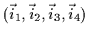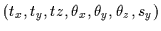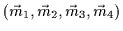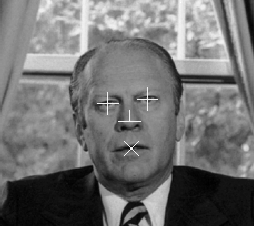Next: P3P - Fitting the Up: Face Normalization and Recognition Previous: Generating the Average 3D

# Inverse 3D Projection

It is essential to fit the 3D range data of the mean face so that it models a face found in a 2D image. Assume we have a 2D image with the coordinates of the eyes, the nose and the mouth perfectly pinpointed, as in Figure. Denote the 2D positions of the eyes, nose and mouth as. The parameters of the 3D modelmust be tuned to align its 3D anchor pointsso that their 2D projections coincide with the set of 2D anchor points. Note that the alignment to the destination pointsinvolves minimizing 8 distances since each of these points is a 2D position. We observe that Equation, which only has 7 degrees of freedom, is over-specified. Thus, there is usually no exact solution to the fitting problem, only approximations.Tony Jebara
2000-06-23